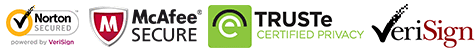FedEx will stop during Oct 1-8
FedEx will stop during Oct 1-8# Astroworld Various Smile Earth Patches For Custom Air Force 1, Perfect Patches For Custom Sneakers/Vans/AF1 Travis Sccot Theme, Best Gift For Her

Regular price \$15.00 \$9.90 Save \$5.10

ONLY 199994 LEFT

 Hours   Minutes   Seconds

Sale Ends Once The Timer Hits Zero!

ONLY 199994 LEFT

Get the easy use astroworld patches to custom your own astroworld AF1 or Vans. Easy use to DIY and make perfect gift for her.

<<<<<<<<<<<<<<<<<<<<<<<<<<<<<<<<<<<<<<<<<<<<<<<<<<<<<<<<

### Why use these astroworld patches:

1. Perfect sizes for sneakers: AF1, Vans, Converse or other shoes.

2. Also can be used on cloth, socks. hoodies, jeans, tshirt....

3. Durable, waterproof and flexible.

4. Easy use, just need to iron on top.

5. Get these astroworld patches to DIY, save lot's money to buy astroworld shoes.

<<<<<<<<<<<<<<<<<<<<<<<<<<<<<<<<<<<<<<<<<<<<<<<<<<<<<<<<

The set includes: 2 large smile earth 2 astroworld 2 sheets of "wish you were here"

The shoes are not included in the set.

<<<<<<<<<<<<<<<<<<<<<<<<<<<<<<<<<<<<<<<<<<<<<<<<<<<<<<<

### Sizes

large smile earth:

Width 4 cm, 1.57 inches;

Height: 4 cm, 1.57 inches

"ASTROWORLD":

Width 10.5 cm, 4.13inches

Height: 1.4 cm, 0.55 inches

"wish you were here":

Width 2.2 cm, 0.87 inches

Height: 2.5 cm, 0.98 inches

<<<<<<<<<<<<<<<<<<<<<<<<<<<<<<<<<<<<<<<<<<<<<<<<<<<<<<<

### How to use:

1. Position the smile earth patches on the shoes with tape

2. Iron on the top of the smile earth, please keep temperature 130-150℃

3. peel the vinyl, the smile earth patches will stick on the shoes.

4. If use normal iron, please turn off the steam, keep the temperature above, use the tip and be careful and patient, for it's heavy to control, better use the mini iron.

<<<<<<<<<<<<<<<<<<<<<<<<<<<<<<<<<<<<<<<<<<<<<<<<<<<<<<<

### Free shipping

people are currently looking at this product

Estimated delivery time 7-15 days

Guaranteed Safe Checkout